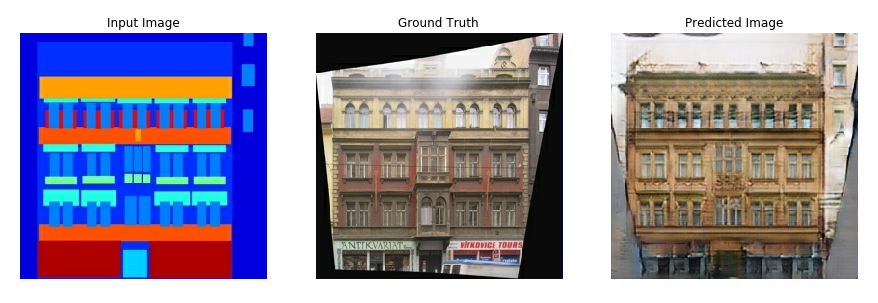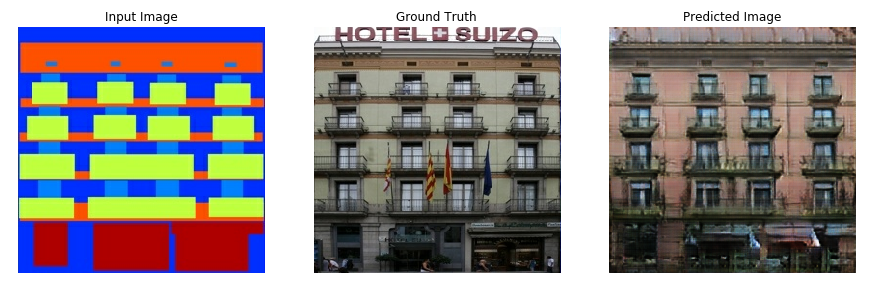# شبکه‌ی مولد تخاصمی pix2pix¶

کدها برگرفته از مثال‌های مخزن گیت‌هاب تنسرفلو است.

https://github.com/tensorflow/tensorflow/tree/master/tensorflow/contrib/eager/python/examples/pix2pix/pix2pix_eager.ipynb

توصیه می‌شود قبل از این نوت‌بوک حتما نوت بوک

55-simple-GAN.ipynb

و

56-DCGAN.ipynb

را مرور کنید.

This notebook demonstrates image to image translation using conditional GAN's, as described in Image-to-Image Translation with Conditional Adversarial Networks. Using this technique we can colorize black and white photos, convert google maps to google earth, etc. Here, we convert building facades to real buildings. We use tf.keras and eager execution to achieve this.

In example, we will use the CMP Facade Database, helpfully provided by the Center for Machine Perception at the Czech Technical University in Prague. To keep our example short, we will use a preprocessed copy of this dataset, created by the authors of the paper above.

Each epoch takes around 58 seconds on a single P100 GPU.

Below is the output generated after training the model for 200 epochs.## Import TensorFlow and enable eager execution¶

In :
# Import TensorFlow >= 1.10 and enable eager execution
import tensorflow as tf
tf.enable_eager_execution()

import os
import time
import numpy as np
import matplotlib.pyplot as plt
import PIL
from IPython.display import clear_output


You can download this dataset and similar datasets from here. As mentioned in the paper we apply random jittering and mirroring to the training dataset.

• In random jittering, the image is resized to 286 x 286 and then randomly cropped to 256 x 256
• In random mirroring, the image is randomly flipped horizontally i.e left to right.
In :
path_to_zip = tf.keras.utils.get_file('facades.tar.gz',
cache_subdir=os.path.abspath('.'),
extract=True)


In :
BUFFER_SIZE = 400
BATCH_SIZE = 1
IMG_WIDTH = 256
IMG_HEIGHT = 256

In :
def load_image(image_file, is_train):
image = tf.image.decode_jpeg(image)

w = tf.shape(image)

w = w // 2
real_image = image[:, :w, :]
input_image = image[:, w:, :]

input_image = tf.cast(input_image, tf.float32)
real_image = tf.cast(real_image, tf.float32)

if is_train:
# random jittering

# resizing to 286 x 286 x 3
input_image = tf.image.resize_images(input_image, [286, 286],
align_corners=True,
method=tf.image.ResizeMethod.NEAREST_NEIGHBOR)
real_image = tf.image.resize_images(real_image, [286, 286],
align_corners=True,
method=tf.image.ResizeMethod.NEAREST_NEIGHBOR)

# randomly cropping to 256 x 256 x 3
stacked_image = tf.stack([input_image, real_image], axis=0)
cropped_image = tf.random_crop(stacked_image, size=[2, IMG_HEIGHT, IMG_WIDTH, 3])
input_image, real_image = cropped_image, cropped_image

if np.random.random() > 0.5:
# random mirroring
input_image = tf.image.flip_left_right(input_image)
real_image = tf.image.flip_left_right(real_image)
else:
input_image = tf.image.resize_images(input_image, size=[IMG_HEIGHT, IMG_WIDTH],
align_corners=True, method=2)
real_image = tf.image.resize_images(real_image, size=[IMG_HEIGHT, IMG_WIDTH],
align_corners=True, method=2)

# normalizing the images to [-1, 1]
input_image = (input_image / 127.5) - 1
real_image = (real_image / 127.5) - 1

return input_image, real_image


## Use tf.data to create batches, map(do preprocessing) and shuffle the dataset¶

In :
train_dataset = tf.data.Dataset.list_files(PATH+'train/*.jpg')
train_dataset = train_dataset.shuffle(BUFFER_SIZE)
train_dataset = train_dataset.map(lambda x: load_image(x, True))
train_dataset = train_dataset.batch(1)

In :
test_dataset = tf.data.Dataset.list_files(PATH+'test/*.jpg')
test_dataset = test_dataset.map(lambda x: load_image(x, False))
test_dataset = test_dataset.batch(1)


## Write the generator and discriminator models¶

• Generator

• The architecture of generator is a modified U-Net.
• Each block in the encoder is (Conv -> Batchnorm -> Leaky ReLU)
• Each block in the decoder is (Transposed Conv -> Batchnorm -> Dropout(applied to the first 3 blocks) -> ReLU)
• There are skip connections between the encoder and decoder (as in U-Net).
• Discriminator

• The Discriminator is a PatchGAN.
• Each block in the discriminator is (Conv -> BatchNorm -> Leaky ReLU)
• The shape of the output after the last layer is (batch_size, 30, 30, 1)
• Each 30x30 patch of the output classifies a 70x70 portion of the input image (such an architecture is called a PatchGAN).
• Input image and the target image, which it should classify as real.
• Input image and the generated image (output of generator), which it should classify as fake.
• We concatenate these 2 inputs together in the code (tf.concat([inp, tar], axis=-1))
• Shape of the input travelling through the generator and the discriminator is in the comments in the code.

In :
OUTPUT_CHANNELS = 3

In :
class Downsample(tf.keras.Model):

def __init__(self, filters, size, apply_batchnorm=True):
super(Downsample, self).__init__()
self.apply_batchnorm = apply_batchnorm
initializer = tf.random_normal_initializer(0., 0.02)

self.conv1 = tf.keras.layers.Conv2D(filters,
(size, size),
strides=2,
kernel_initializer=initializer,
use_bias=False)
if self.apply_batchnorm:
self.batchnorm = tf.keras.layers.BatchNormalization()

def call(self, x, training):
x = self.conv1(x)
if self.apply_batchnorm:
x = self.batchnorm(x, training=training)
x = tf.nn.leaky_relu(x)
return x

class Upsample(tf.keras.Model):

def __init__(self, filters, size, apply_dropout=False):
super(Upsample, self).__init__()
self.apply_dropout = apply_dropout
initializer = tf.random_normal_initializer(0., 0.02)

self.up_conv = tf.keras.layers.Conv2DTranspose(filters,
(size, size),
strides=2,
kernel_initializer=initializer,
use_bias=False)
self.batchnorm = tf.keras.layers.BatchNormalization()
if self.apply_dropout:
self.dropout = tf.keras.layers.Dropout(0.5)

def call(self, x1, x2, training):
x = self.up_conv(x1)
x = self.batchnorm(x, training=training)
if self.apply_dropout:
x = self.dropout(x, training=training)
x = tf.nn.relu(x)
x = tf.concat([x, x2], axis=-1)
return x

class Generator(tf.keras.Model):

def __init__(self):
super(Generator, self).__init__()
initializer = tf.random_normal_initializer(0., 0.02)

self.down1 = Downsample(64, 4, apply_batchnorm=False)
self.down2 = Downsample(128, 4)
self.down3 = Downsample(256, 4)
self.down4 = Downsample(512, 4)
self.down5 = Downsample(512, 4)
self.down6 = Downsample(512, 4)
self.down7 = Downsample(512, 4)
self.down8 = Downsample(512, 4)

self.up1 = Upsample(512, 4, apply_dropout=True)
self.up2 = Upsample(512, 4, apply_dropout=True)
self.up3 = Upsample(512, 4, apply_dropout=True)
self.up4 = Upsample(512, 4)
self.up5 = Upsample(256, 4)
self.up6 = Upsample(128, 4)
self.up7 = Upsample(64, 4)

self.last = tf.keras.layers.Conv2DTranspose(OUTPUT_CHANNELS,
(4, 4),
strides=2,
kernel_initializer=initializer)

@tf.contrib.eager.defun
def call(self, x, training):
# x shape == (bs, 256, 256, 3)
x1 = self.down1(x, training=training) # (bs, 128, 128, 64)
x2 = self.down2(x1, training=training) # (bs, 64, 64, 128)
x3 = self.down3(x2, training=training) # (bs, 32, 32, 256)
x4 = self.down4(x3, training=training) # (bs, 16, 16, 512)
x5 = self.down5(x4, training=training) # (bs, 8, 8, 512)
x6 = self.down6(x5, training=training) # (bs, 4, 4, 512)
x7 = self.down7(x6, training=training) # (bs, 2, 2, 512)
x8 = self.down8(x7, training=training) # (bs, 1, 1, 512)

x9 = self.up1(x8, x7, training=training) # (bs, 2, 2, 1024)
x10 = self.up2(x9, x6, training=training) # (bs, 4, 4, 1024)
x11 = self.up3(x10, x5, training=training) # (bs, 8, 8, 1024)
x12 = self.up4(x11, x4, training=training) # (bs, 16, 16, 1024)
x13 = self.up5(x12, x3, training=training) # (bs, 32, 32, 512)
x14 = self.up6(x13, x2, training=training) # (bs, 64, 64, 256)
x15 = self.up7(x14, x1, training=training) # (bs, 128, 128, 128)

x16 = self.last(x15) # (bs, 256, 256, 3)
x16 = tf.nn.tanh(x16)

return x16

In :
class DiscDownsample(tf.keras.Model):

def __init__(self, filters, size, apply_batchnorm=True):
super(DiscDownsample, self).__init__()
self.apply_batchnorm = apply_batchnorm
initializer = tf.random_normal_initializer(0., 0.02)

self.conv1 = tf.keras.layers.Conv2D(filters,
(size, size),
strides=2,
kernel_initializer=initializer,
use_bias=False)
if self.apply_batchnorm:
self.batchnorm = tf.keras.layers.BatchNormalization()

def call(self, x, training):
x = self.conv1(x)
if self.apply_batchnorm:
x = self.batchnorm(x, training=training)
x = tf.nn.leaky_relu(x)
return x

class Discriminator(tf.keras.Model):

def __init__(self):
super(Discriminator, self).__init__()
initializer = tf.random_normal_initializer(0., 0.02)

self.down1 = DiscDownsample(64, 4, False)
self.down2 = DiscDownsample(128, 4)
self.down3 = DiscDownsample(256, 4)

# we are zero padding here with 1 because we need our shape to
# go from (batch_size, 32, 32, 256) to (batch_size, 31, 31, 512)
self.conv = tf.keras.layers.Conv2D(512,
(4, 4),
strides=1,
kernel_initializer=initializer,
use_bias=False)
self.batchnorm1 = tf.keras.layers.BatchNormalization()

# shape change from (batch_size, 31, 31, 512) to (batch_size, 30, 30, 1)
self.last = tf.keras.layers.Conv2D(1,
(4, 4),
strides=1,
kernel_initializer=initializer)

@tf.contrib.eager.defun
def call(self, inp, tar, training):
# concatenating the input and the target
x = tf.concat([inp, tar], axis=-1) # (bs, 256, 256, channels*2)
x = self.down1(x, training=training) # (bs, 128, 128, 64)
x = self.down2(x, training=training) # (bs, 64, 64, 128)
x = self.down3(x, training=training) # (bs, 32, 32, 256)

x = self.zero_pad1(x) # (bs, 34, 34, 256)
x = self.conv(x)      # (bs, 31, 31, 512)
x = self.batchnorm1(x, training=training)
x = tf.nn.leaky_relu(x)

x = self.zero_pad2(x) # (bs, 33, 33, 512)
# don't add a sigmoid activation here since
# the loss function expects raw logits.
x = self.last(x)      # (bs, 30, 30, 1)

return x

In :
# The call function of Generator and Discriminator have been decorated
# with tf.contrib.eager.defun()
# We get a performance speedup if defun is used (~25 seconds per epoch)
generator = Generator()
discriminator = Discriminator()


## Define the loss functions and the optimizer¶

• Discriminator loss

• The discriminator loss function takes 2 inputs; real images, generated images
• real_loss is a sigmoid cross entropy loss of the real images and an array of ones(since these are the real images)
• generated_loss is a sigmoid cross entropy loss of the generated images and an array of zeros(since these are the fake images)
• Then the total_loss is the sum of real_loss and the generated_loss
• Generator loss

• It is a sigmoid cross entropy loss of the generated images and an array of ones.
• The paper also includes L1 loss which is MAE (mean absolute error) between the generated image and the target image.
• This allows the generated image to become structurally similar to the target image.
• The formula to calculate the total generator loss = gan_loss + LAMBDA * l1_loss, where LAMBDA = 100. This value was decided by the authors of the paper.
In :
LAMBDA = 100

In :
def discriminator_loss(disc_real_output, disc_generated_output):
real_loss = tf.losses.sigmoid_cross_entropy(multi_class_labels = tf.ones_like(disc_real_output),
logits = disc_real_output)
generated_loss = tf.losses.sigmoid_cross_entropy(multi_class_labels = tf.zeros_like(disc_generated_output),
logits = disc_generated_output)

total_disc_loss = real_loss + generated_loss


In :
def generator_loss(disc_generated_output, gen_output, target):
gan_loss = tf.losses.sigmoid_cross_entropy(multi_class_labels = tf.ones_like(disc_generated_output),
logits = disc_generated_output)
# mean absolute error
l1_loss = tf.reduce_mean(tf.abs(target - gen_output))

total_gen_loss = gan_loss + (LAMBDA * l1_loss)


In :
generator_optimizer = tf.train.AdamOptimizer(2e-4, beta1=0.5)


## Checkpoints (Object-based saving)¶

In :
checkpoint_dir = './training_checkpoints'
checkpoint_prefix = os.path.join(checkpoint_dir, "ckpt")
checkpoint = tf.train.Checkpoint(generator_optimizer=generator_optimizer,
discriminator_optimizer=discriminator_optimizer,
generator=generator,
discriminator=discriminator)


## Training¶

• We start by iterating over the dataset
• The generator gets the input image and we get a generated output.
• The discriminator receives the input_image and the generated image as the first input. The second input is the input_image and the target_image.
• Next, we calculate the generator and the discriminator loss.
• Then, we calculate the gradients of loss with respect to both the generator and the discriminator variables(inputs) and apply those to the optimizer.

## Generate Images¶

• After training, its time to generate some images!
• We pass images from the test dataset to the generator.
• The generator will then translate the input image into the output we expect.
• Last step is to plot the predictions and voila!
In :
EPOCHS = 200

In :
def generate_images(model, test_input, tar):
# the training=True is intentional here since
# we want the batch statistics while running the model
# on the test dataset. If we use training=False, we will get
# the accumulated statistics learned from the training dataset
# (which we don't want)
prediction = model(test_input, training=True)
plt.figure(figsize=(15,15))

display_list = [test_input, tar, prediction]
title = ['Input Image', 'Ground Truth', 'Predicted Image']

for i in range(3):
plt.subplot(1, 3, i+1)
plt.title(title[i])
# getting the pixel values between [0, 1] to plot it.
plt.imshow(display_list[i] * 0.5 + 0.5)
plt.axis('off')
plt.show()

In :
def train(dataset, epochs):
for epoch in range(epochs):
start = time.time()

for input_image, target in dataset:

gen_output = generator(input_image, training=True)

disc_real_output = discriminator(input_image, target, training=True)
disc_generated_output = discriminator(input_image, gen_output, training=True)

gen_loss = generator_loss(disc_generated_output, gen_output, target)
disc_loss = discriminator_loss(disc_real_output, disc_generated_output)

generator.variables)
discriminator.variables)

generator.variables))
discriminator.variables))

if epoch % 1 == 0:
clear_output(wait=True)
for inp, tar in test_dataset.take(1):
generate_images(generator, inp, tar)

# saving (checkpoint) the model every 20 epochs
if (epoch + 1) % 20 == 0:
checkpoint.save(file_prefix = checkpoint_prefix)

print ('Time taken for epoch {} is {} sec\n'.format(epoch + 1,
time.time()-start))

In :
train(train_dataset, EPOCHS)


## Restore the latest checkpoint and test¶

In :
# restoring the latest checkpoint in checkpoint_dir
checkpoint.restore(tf.train.latest_checkpoint(checkpoint_dir))


## Testing on the entire test dataset¶

In :
# Run the trained model on the entire test dataset
for inp, tar in test_dataset:
generate_images(generator, inp, tar)

دانشگاه تربیت دبیر شهید رجایی
مباحث ویژه 2 - یادگیری عمیق پیشرفته
علیرضا اخوان پور
97-98
SRTTU.edu - Class.Vision - AkhavanPour.ir
In :